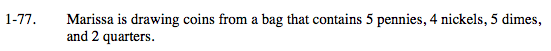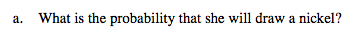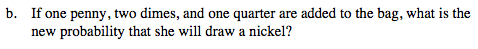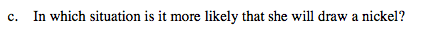### Home > MC2 > Chapter 1 > Lesson 1.2.3 > Problem1-77

1-77.Remember, probability is the number of successful outcomes out of the total number of possible outcomes.

How many nickels are in the bag?
How many total coins are in the bag?

$\frac{4}{16}= \frac{1}{4}$What is the new number of nickels?
What is the new total number of coins?